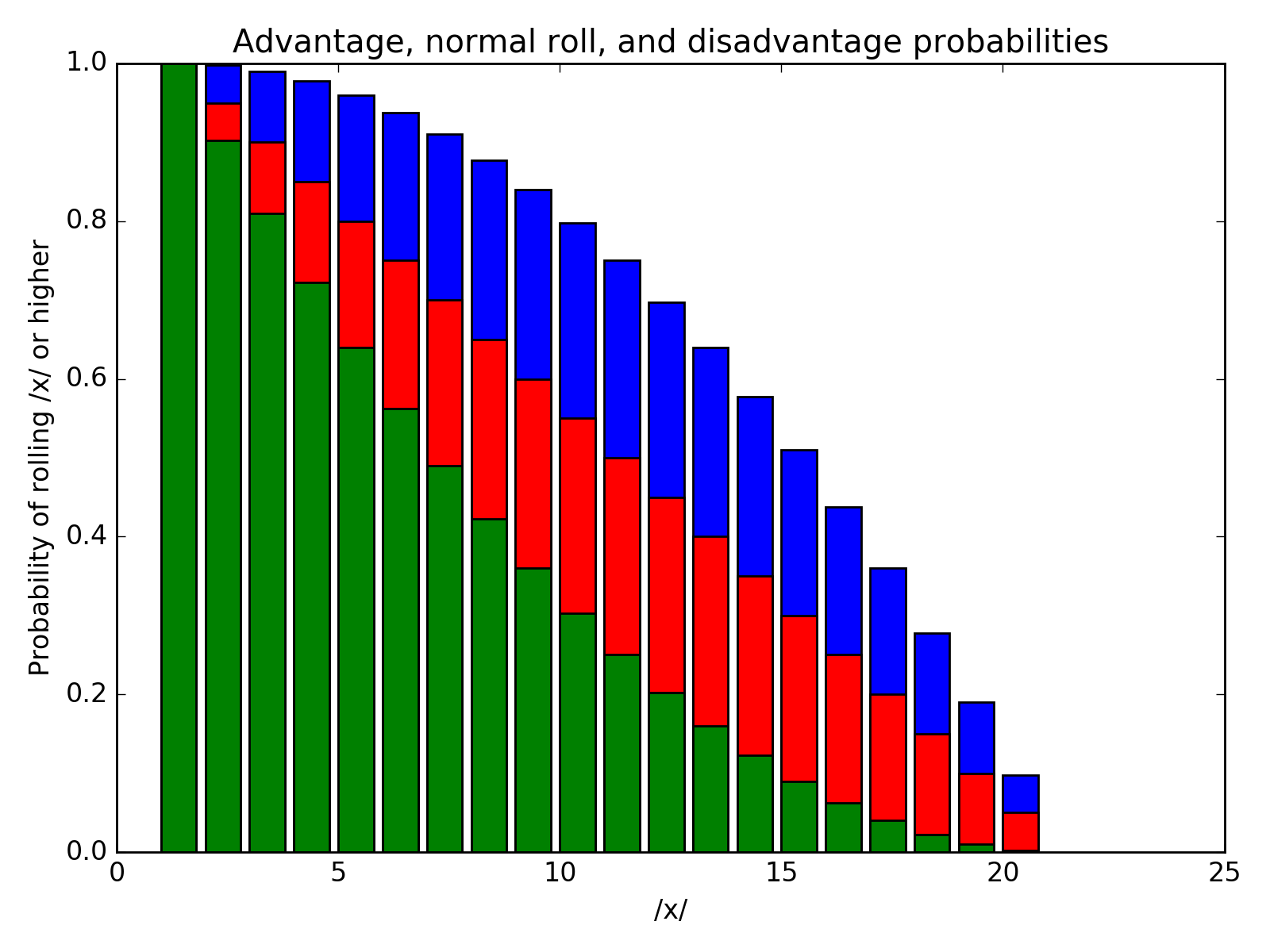# D&D Probability distributions

## 1 Dice averages

 D4 D6 D8 D10 D12 D20 2.5 3.5 4.5 5.5 6.5 10.5

A normal, unweighted die has equal probabilities, so the cumulative probability distribution is just a straight line. However, the advantaged throws are much better, having

import numpy as np

d20 = np.arange(1,21)

normal_roll = (20-d20)/20.0
return np.array([d20.tolist(),
normal_roll.tolist(),
.transpose()

 1 0.9975 0.95 0.9025 2 0.99 0.9 0.81 3 0.9775 0.85 0.7225 4 0.96 0.8 0.64 5 0.9375 0.75 0.5625 6 0.91 0.7 0.49 7 0.8775 0.65 0.4225 8 0.84 0.6 0.36 9 0.7975 0.55 0.3025 10 0.75 0.5 0.25 11 0.6975 0.45 0.2025 12 0.64 0.4 0.16 13 0.5775 0.35 0.1225 14 0.51 0.3 0.09 15 0.4375 0.25 0.0625 16 0.36 0.2 0.04 17 0.2775 0.15 0.0225 18 0.19 0.1 0.01 19 0.0975 0.05 0.0025 20 0 0 0
import numpy as np
import matplotlib.pyplot as plot

rollsNP = np.array(rolls)

fig, ax = plot.subplots()

d20 = np.arange(1,21)

ax.set_xlabel("/x/")
ax.set_ylabel("Probability of rolling /x/ or higher")

plot.bar(rollsNP.T, rollsNP.T, color='red', label="normal roll")

fig.tight_layout()
fig.savefig(name, dpi=200)
return name## 3 Multiple rolls

Binomial distribution

 pmf $${n \choose k}\,p^{k}(1-p)^{n-k}$$ CDF $$\sum^{\lfloor k \rfloor}_{i=0} {n \choose i} p^i {(1-p)}^{n-i}$$ Mean $$np$$ Median $$\lfloor np\rfloor \text{ or } \lceil np\rceil$$ Mode $$\lfloor (n+1)p\rfloor \text { or } \lceil (n+1)p\rceil -1$$ Variance $$np(1-p)$$

were $${n\choose k} = \frac{n!}{k!(n-k)!}$$ is the binomial coefficient (nCk on calculators), also known as choosing $$k$$ subsets out of $$n$$ elements.

Created: 2018-10-04 Thu 18:52

Validate